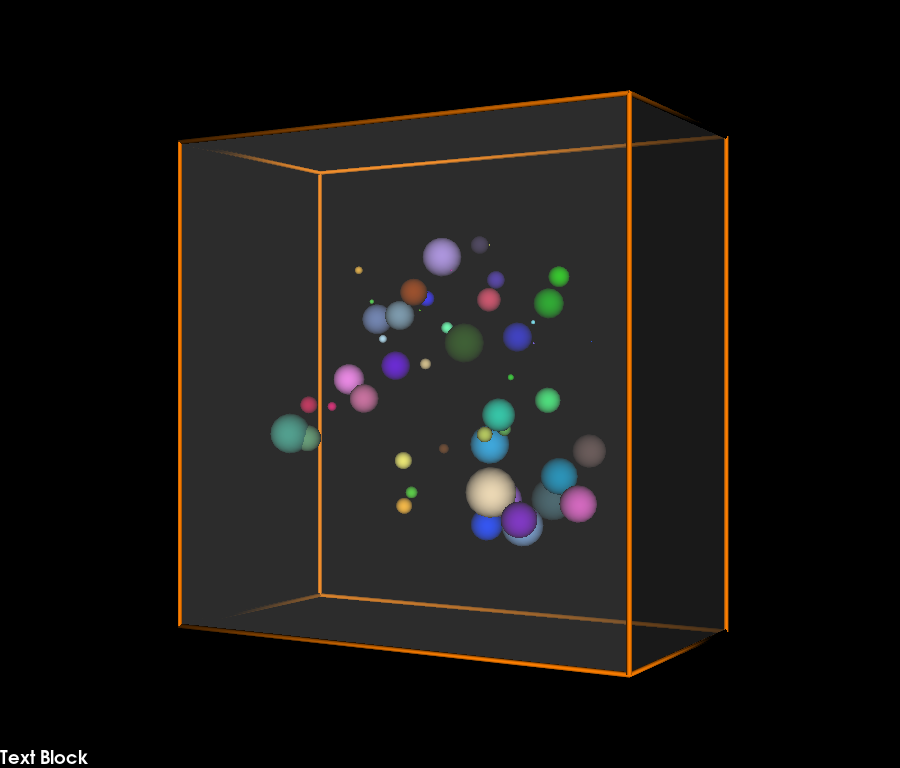# Collisions of particles in a box#

This is a simple demonstration of how you can simulate moving particles in a box using FURY.

In this example, the particles collide with each other and with the walls of the container. When the collision happens between two particles, the particle with less velocity changes its color and gets the same color as the particle with higher velocity. For simplicity, in this demo we do not apply forces.

```import itertools

import numpy as np

from fury import actor, ui, utils, window
```

Here, we define the edges of the box.

```def box_edges(box_lx, box_ly, box_lz):

edge1 = 0.5 * np.array(
[
[box_lx, box_ly, box_lz],
[box_lx, box_ly, -box_lz],
[-box_lx, box_ly, -box_lz],
[-box_lx, box_ly, box_lz],
[box_lx, box_ly, box_lz],
]
)
edge2 = 0.5 * np.array([[box_lx, box_ly, box_lz], [box_lx, -box_ly, box_lz]])
edge3 = 0.5 * np.array([[box_lx, box_ly, -box_lz], [box_lx, -box_ly, -box_lz]])
edge4 = 0.5 * np.array([[-box_lx, box_ly, -box_lz], [-box_lx, -box_ly, -box_lz]])
edge5 = 0.5 * np.array([[-box_lx, box_ly, box_lz], [-box_lx, -box_ly, box_lz]])
lines = [edge1, -edge1, edge2, edge3, edge4, edge5]
return lines
```

Here we define collision between walls-particles and particle-particle. When collision happens, the particle with lower velocity gets the color of the particle with higher velocity

```def collision():
global xyz
num_vertices = vertices.shape
sec = int(num_vertices / num_particles)

for i, j in np.ndindex(num_particles, num_particles):

if i == j:
continue
distance = np.linalg.norm(xyz[i] - xyz[j])
vel_mag_i = np.linalg.norm(vel[i])
vel_mag_j = np.linalg.norm(vel[j])
# Collision happens if the distance between the centers of two
# particles is less or equal to the sum of their radii
vel[i] = -vel[i]
vel[j] = -vel[j]
if vel_mag_j > vel_mag_i:
vcolors[i * sec : i * sec + sec] = vcolors[j * sec : j * sec + sec]
if vel_mag_i > vel_mag_j:
vcolors[j * sec : j * sec + sec] = vcolors[i * sec : i * sec + sec]
xyz[i] = xyz[i] + vel[i] * dt
xyz[j] = xyz[j] + vel[j] * dt
# Collision between particles-walls;
vel[:, 0] = np.where(
(
(xyz[:, 0] <= -0.5 * box_lx + radii[:])
| (xyz[:, 0] >= (0.5 * box_lx - radii[:]))
),
-vel[:, 0],
vel[:, 0],
)
vel[:, 1] = np.where(
(
(xyz[:, 1] <= -0.5 * box_ly + radii[:])
| (xyz[:, 1] >= (0.5 * box_ly - radii[:]))
),
-vel[:, 1],
vel[:, 1],
)
vel[:, 2] = np.where(
(
(xyz[:, 2] <= -0.5 * box_lz + radii[:])
| (xyz[:, 2] >= (0.5 * box_lz - radii[:]))
),
-vel[:, 2],
vel[:, 2],
)
```

We define position, velocity, color and radius randomly for 50 particles inside the box.

```global xyz
num_particles = 50
box_lx = 20
box_ly = 20
box_lz = 10
steps = 1000
dt = 0.05
xyz = (
np.array([box_lx, box_ly, box_lz]) * (np.random.rand(num_particles, 3) - 0.5) * 0.6
)
vel = 4 * (np.random.rand(num_particles, 3) - 0.5)
colors = np.random.rand(num_particles, 3)
```

With box, streamtube and sphere actors, we can create the box, the edges of the box and the spheres respectively.

```scene = window.Scene()
box_centers = np.array([[0, 0, 0]])
box_directions = np.array([[0, 1, 0]])
box_colors = np.array([[1, 1, 1, 0.2]])
box_actor = actor.box(
box_centers, box_directions, box_colors, scales=(box_lx, box_ly, box_lz)
)

lines = box_edges(box_lx, box_ly, box_lz)
line_actor = actor.streamtube(lines, colors=(1, 0.5, 0), linewidth=0.1)

showm = window.ShowManager(
scene, size=(900, 768), reset_camera=True, order_transparent=True
)

tb = ui.TextBlock2D(bold=True)
scene.zoom(0.8)
scene.azimuth(30)

# use itertools to avoid global variables
counter = itertools.count()

vertices = utils.vertices_from_actor(sphere_actor)
vcolors = utils.colors_from_actor(sphere_actor, 'colors')
no_vertices_per_sphere = len(vertices) / num_particles
initial_vertices = vertices.copy() - np.repeat(xyz, no_vertices_per_sphere, axis=0)

def timer_callback(_obj, _event):
global xyz
cnt = next(counter)
tb.message = "Let's count up to 1000 and exit :" + str(cnt)
xyz = xyz + vel * dt
collision()

vertices[:] = initial_vertices + np.repeat(xyz, no_vertices_per_sphere, axis=0)
utils.update_actor(sphere_actor)

scene.reset_clipping_range()
showm.render()

if cnt == steps:
showm.exit()